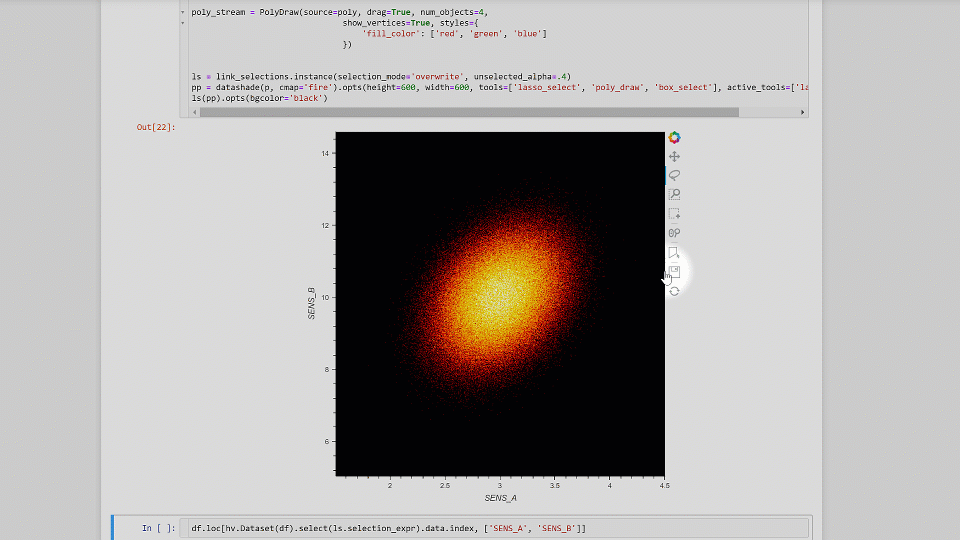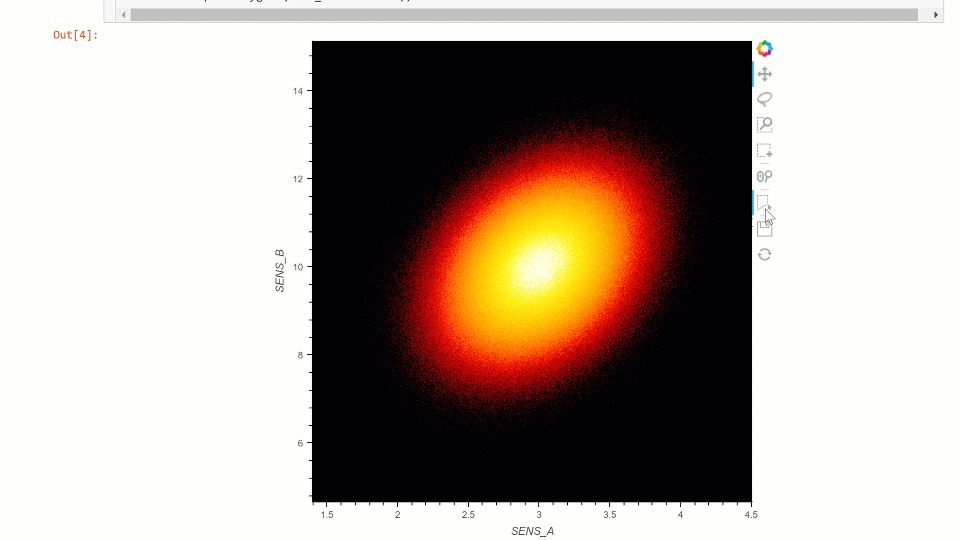# Holoviews spatialpandas lasso or polygon_draw select on shaded points plot

Hi,
I am making shaded points plots and then doing selections on top. It is a great tool! It turns out that the selects on shaded points plots works nicely and fast using box select. But I also would really like to use lasso_select and ideally polygon_draw (as it would produce less points than lasso). When I do lasso_select it turns out that the selection contains nan values from the series which is not expected. This seems only to happen when spatialpandas is used as backend intersect engine. Shapely work ‘correctly’ it seems, but is much slower.
Is there anything I might do in order to resolve this?

One question relating to performance: I was wondering if the intersect on the datashaded point charts is working on the aggregate grid or on the actual vector points vs. polygon?

Adding an animated gif that shows that nan’s are selected in my current example.Code example below:

``````%load_ext autoreload

import numpy as np
import pandas as pd
import hvplot.pandas

# Import plotting libs
import holoviews as hv
from holoviews.streams import PolyDraw
from holoviews import opts

# set bakend
hv.extension('bokeh')
``````

Create dataset:

``````# Prepare data

np.random.seed(1)
n = 10_000_000
n1 = n - 3_000_000
n2 = n - n1
ids = n1 * ['ONE'] +  n2 * ['TWO']

data = np.random.multivariate_normal((3,10), [[0.1, 0.1], [0.1, 1.0]], (n,))

SENS_A = data[:,0]
SENS_B = data[:,1]

# Make holes in data
SENS_A = np.where(np.random.rand(n) > .5, np.NaN, SENS_A)
SENS_B = np.where(np.random.rand(n) < .2, np.NaN, SENS_B)

data_dict = {'id': ids,
'SENS_A': SENS_A,
'SENS_B': SENS_B}

df = pd.DataFrame(data_dict)

# Ensure there are nan's
try:
display(df.isna().sum())
except:
pass
``````

``````p = hv.Points(df, kdims=['SENS_A', 'SENS_B'])

pp = datashade(p, cmap='fire').opts(height=600, width=600, tools=['lasso_select', 'poly_select', 'box_select'], active_tools=['lasso_select'])
ls(pp).opts(bgcolor='black')
``````

Do interactive lasso selection in plot and then display the selected data:

``````df.loc[hv.Dataset(df).select(ls.selection_expr).data.index, ['SENS_A', 'SENS_B']]
``````

Ensure that the values selected are not containing nans from any of the dimensions.

``````n_selected_rows_with_any_nan = df.loc[hv.Dataset(df).select(ls.selection_expr).data.index, ['SENS_A', 'SENS_B']].isna().any(axis=1).sum()
criterium = (n_selected_rows_with_any_nan == 0)
assert_msg = "Selected points exist in 2d space and should by definition not have any nan's"

assert criterium, assert_msg
``````

Library versions:
holoviews: version: 1.14.4
param: version: 1.10.1
bokeh: version: 2.3.2
panel: version: 0.11.1
spatialpandas: version: 0.4.3
shapely: version: 1.7.1

Update:
When I use ‘poly_select’ as a tool it works nicely. (But, I do not see so well my polygon vertices while drawing, will dig into how to control that a bit better).
I updated the code snippet to use 10 mill records which is bit closer to larger real world datasets I often work with. Thus I am eager at exploring if the workings of datashader can come into play to facilitate the points in sample-bucket in polygon overlays (if not already happening) . The below animation is edited to move away waiting time.I am left with the following findings and challenges:

• ‘lasso_select’ seems to return wrong results when ‘spatialpandas’ is doing points in polygon intersect (potential bug)
• ‘poly_select’ works correctly for the points in polygon select
• for both ‘lasso_select’ and ‘poly_select’ (i.e. polygon selects) it would be beneficial to somehow make the GUI and more responsive (faster) and more clear (draw vertixes an lines imediately when clicking with control on styling) with datasets larger that 10 million rows.
• for polygon selects towards the input dataframe it would be fantastic if someone knows how to speed up the intersect operation there too…is there anything to gain?